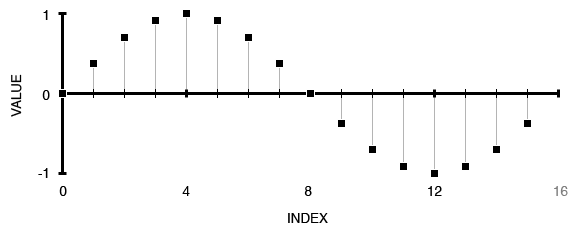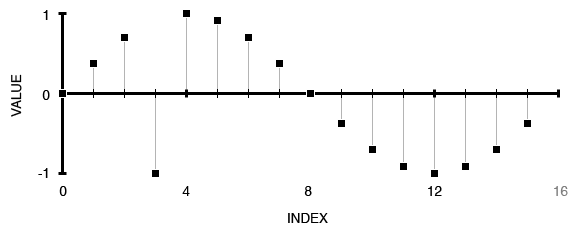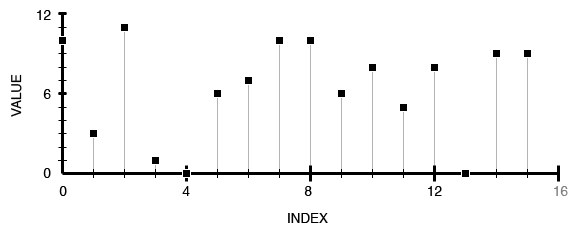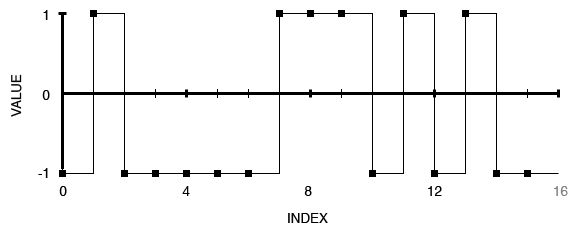## Introduction

Csound comes with a variety of GEN routines for generating data that is stored in function tables. Common uses include single-cycle waveforms, the values of a steps sequencer, the contents of a file, the shape of an envelope, noise, etc.

Though the options are vast, the built-in GEN routines do not accommodate every possible scenario. Csound is equipped with all the necessary elements for composers and digital-synthesizer makers to design and implement their own instrument-based GEN routines.

Examples from Article: GEN_Instruments.zip

## I. Background

In this section, I will cover some of the basics of ftables and GEN routines.

### What is a Function Table?

A Csound function table (ftable) is a data structure for storing a list of floating-point numbers in memory. An ftable is analogous to a single dimension array . Each value, also known as an element, is stored at a particular location called an index. Indices are referred to by whole numbers. Function tables are also known as lookup tables .

The common Csound practice is to declare the size of a table with a value that is a power of 2, or power of 2 plus 1. Non power of 2 sizes can also be used, but must be specified as negative values. The minimum size of an ftable is 2, while the maximum is 16777216 .

Function tables are created using the f statement in the score, or using the ftable line of opcodes in the orchestra. The data that is stored in a table is usually computed with a GEN routine, though it can be gathered by other means, such as using a tabw_i opcode in an instrument.

### What is a GEN routine?

A GEN routine is a method for placing values into an ftable. The values may be generated by an algorithm, read from a file, read from an existing table, or by other means. When an ftable is created, a user specifies which GEN routine will be used.

Csound comes preloaded with 38+ routines. Routines include, but are not limited to, reading and storing a sound file, pre-calculating a stored waveform, specifying individual points of data and generating random numbers.

As an example, GEN10 generates a composite waveforms made up of weighted sums of simple sinusoids . The f statement in Figure 1(a) generates a 16-point sine wave utilizing GEN10. Figure 1(b) shows the values generated alongside their related indices. Figure 1(c) shows the data plotted onto a graph, revealing the shape of a sine wave.

(a)

`f 1 0 16 10 1`

(b)

```0:   0.000000
1:   0.382683
2:   0.707107
3:   0.923880
4:   1.000000
5:   0.923880
6:   0.707107
7:   0.382683
8:   0.000000
9:  -0.382683
10: -0.707107
11: -0.923880
12: -1.000000
13: -0.923880
14: -0.707107
15: -0.382683
```

(c)Figure 1. A 16 point sine wave. See sine_16.csd: (a) Sine table created in score with GEN10; (b) Index-value pairs for each element; (c) Table plotted onto a graph.

GEN routines have an extensive history, going as far back as MUSIC V, if not farther. In The Technology of Computer Music, the question is asked, why go to all the trouble of having a GEN program compute and store numbers and then have the OSC program modify and repeat these numbers? Efficiency is the answer. Back then, the cost of continuously calculating a basic sine wave was quite expensive. To alleviate the strain on the CPU, and to reduce render times, a single cycle waveform would be generated once and placed into a table in memory. A wavetable oscillator could then read values from the table, which was faster than the continuous calclucation.

### What is a GEN Instrument?

A GEN instrument is a Csound orchestra implementation of a GEN routine. They are written as instruments and may utilize existing GEN routines, along with custom user-defined opcodes. While built-in GENs are activated with an f statement in the score or ftgen in the orchestra, their instrument counterparts are called upon using an i event in the score, or event_i in the orchestra.

## II. Basic GEN Techniques

In this section, the techniques required to design and implement a basic GEN instrument are covered. Though the requirements for any particular GEN instrument can vary greatly, there are some common basic components, as outlined here:

• Assigning Instrument Numbers
• Parameters
• Table Creation
• Looping
• Algorithm
• Turn off

### Assigning Instrument Numbers

When an f statement and an instrument event are scheduled to begin at the same time in the score, the f statement always runs first. This ensures that ftables are created before player instruments try to access them.

Csound instruments are executed from lowest to highest value. In order for GEN instruments to mimic the precedence of the f statement, they must be assigned with instrument numbers lower than any player instruments that access tables created by them.

If a GEN instrument has a higher number assigned to it than a player instrument, and they are both scheduled to begin at the same time, the player instrument will attempt to use an ftable that has not yet been created, or if an ftable already exists at that address, the wrong data will be available to the player instrument. For this reason, it is good practice to place GEN instruments at or near the beginning of the instrument chain, before any player instruments.

```instr 1  ; GEN instrument
...generate a table...
endin

instr 2  ; Player instrument
...uses table created by instr 1...
endin
```

Figure 2. The GEN instrument is properly placed before the player instrument.

### Parameters

GEN Instruments should be written as generally as possible. Function numbers and sizes should not be hard wired to an instrument, in most cases, so they can be reused to create multiple tables. The two parameters found in most GEN instruments are the ftable number and the size of the table.

```ifn = p4
isize = p5```

Figure 3. Function Table number and size receive their values from pfields 4 and 5.

Depending on the circumstances, a GEN instrument may benefit greatly from having additional parameters. The more parameters you implement, the more flexible your GEN will be. You should be aware that there is often a trade off between flexibility and ease of use, as too many parameters may cause the generator to be unwieldy.

### Table Creation

In order to fill a table with numbers, a table must first be created. The best practice for most scenarios is to create an empty table filled with zeros. A GEN routine does not exist for the sole purpose of creating a table padded with zeros. However, there are a few different solutions to this problem. The easiest solution is to use GEN10 and set the strength of the first sinusoid to zero, as shown in Figure 4.

`itemp ftgen ifn, 0, isize, 10, 0`

Figure 4. Creates an ftable padded with zeros utilizing GEN10.
.

There are various opcodes for reading and writing specific elements in a table. This article focuses on two: tab_i and tabw_i. The i signifies that these opcodes work at the initialization stage of an instrument, which is not to be confused with interpolation, another common Csound idiom. These two opcodes were chosen over the alternatives for their ability to access elements quickly. For other possibilities, refer to the section Read/Write Operations in the The Canonical Csound Reference Manual .

To read an individual element, tab_i is used to read a value from a specified index. Figure 5(a) reads the value located at index 3 from table 1, and places it into ivalue. This value is 0.923880.

Writing to a table is accomplished with tabw_i. Figure 5(b) replaces the original value of index 3 in table 1 with -1. Compare the difference between Figure 1(c) with Figure 5(c).

(a)

`ivalue tab_i 3, 1`

(b)

`tabw_i -1.0, 3, 1`

(c)Figure 5. Read and write. See sine_16_rw.csd: (a) Value at index 3 in table 1 is read; (b) Value -1.0 is written to index 3 in table 1; (c) 16 point sine graph from Figure 1 updated to reflect the new value.

### Looping

A loop is used to iterate over every value in an ftable. During each iteration, a value is generated with the algorithm, and stored in the ftable.

```i_index = 0
loop_start:

...generate a value...
tabw_i ivalue, i_index, ifn

loop_lt i_index, 1, isize, loop_start
```

Figure 6. An outline for a loop used for generating and writing data to a table.

For a full explanation on loops and conditional branching in Csound, read Steven Yi's articles "Control Flow Pt. 1" and "Control Flow Pt. 2" .

### Algorithm

The heart of a GEN instrument generally happens within the loop, as this is where a specific algorithm is utilized to generate values for each point in the table. The nature of the numbers generated is defined by the algorithm. An algorithm may simply be a random number generator, or something more complex such as a bandlimited single-cycle waveform generator.

### Turn Off

This last step terminates the current instance of the instrument. Since all the work is done during the initialization period of the instrument, the event can be turned off since there is no work left to do. This is accomplished with the turnoff opcode.

Complex problems often require complex solutions. In this section, I go beyond the basics and cover the following advanced GEN techniques:

• Processing an Existing Table
• Validating Input
• User-Defined Opcodes
• Guard Points
• Plugins

### Processing an Existing Table

Instead of generating data to place into a table, data can be retrieved from an existing table, processed, and then placed into a new table. This requires reading values from a source table, applying a processing algorithm to these values, and then writing them to a destination table. Generally, this happens within the loop of a GEN instrument, as outlined in Figure 7.

```i_index = 0
loop_start:

...process the value...
tabw_i iwrite_value, i_index, idestination_fn

loop_lt i_index, 1, isize, loop_start
```

Figure 7. An outline for a table processor GEN instrument. A value is read from the source table, processed, and placed into the destination table.

There is no requirement that a new table must be created when processing an existing table. There will be times when overwriting values of an existing table is the right design choice.

### Validating Input

To help reduce bugs introduced by the user when creating ftables, GEN instruments can be designed with mechanisms that check the validity of passed pfield values. Validations may be used to check if values fall within an acceptable range, a table exists or not, the size is a power of 2, etc.

Figure 8 demonstrates a simple example where the instrument checks the two ftable numbers against one another. If the two numbers are identical, the instrument writes a warning message to the message window, then turns off the instrument.

```if ifn1 == ifn2 then
prints "WARNING!! pfields 4 and 5 must supply different function\n"
prints "table numbers. Skipping GEN scale.\n"

turnoff
endif
```

Figure 8. Asserts that ifn1 and ifn2 refer to two different tables.

### User-Defined Opcodes

Custom ftable generator and processor engines can be modularized by utilizing user-defined opcodes (UDO). Instead of placing table generator code inside the body of an instrument, it can be placed inside an UDO. This is a good programming practice as UDOs are reusable functions, and using them will reduce potential code duplication and can speed up the development process.

The form of a GEN UDO is very similar to that of a GEN instrument, as seen in Figure 9. However, GEN UDOs cannot be called directly from the score, and must be placed inside a wrapper instrument to make these functions available to the score. A wrapper is a thin interface layer that exposes GEN UDO functionality to the score . Multiple GEN UDOs can be combined into a single GEN instrument, as is the case with the example GEN ScaleAndBias.

(a)

```opcode MyGEN, 0, ii
ifn, isize xin

i_index = 0
itemp ftgen ifn, 0, isize, 10, 0

i_index = 0
loop_start:

...generate a value...
tabw_i ivalue, i_index, ifn

loop_lt i_index, 1, isize, loop_start
endop
```

(b)

```instr 1
ifn = p4
isize = p5

MyGEN ifn, isize

turnoff
endin
```

Figure 9. GEN UDO: (a) The GEN routine resides inside of a user-defined opcode; (b) The instrument acts as a wrapper to the UDO, enabling the GEN to be accessed in the score.

### Guard Points

Certain opcodes utilize a guard point for interpolated lookup. Depending on the context in which you use tables generated from GEN instruments, you may need to add a guard point to your table. The Csound Manual says this about guard points:
For arrays whose length is a power of 2, space allocation always provides for 2n points plus an additional guard point. The guard point value, used during interpolated lookup, can be automatically set to reflect the table's purpose: If size is an exact power of 2, the guard point will be a copy of the first point; this is appropriate for interpolated wrap-around lookup as in oscili, etc., and should even be used for non-interpolating oscil for safe consistency. If size is set to 2 n + 1, the guard point value automatically extends the contour of table values; this is appropriate for single-scan functions such in envplx, oscil1, oscil1i, etc. (The Canonical Csound Reference Manual)

There are two opcodes for writing a table's guard point: tableigpw and tablegpw. For the purposes of this article, the preferred opcode is tableigpw, as the methods described here all work during init-time. To use, simply place the code in Figure 10 at both the end of the loop stage and before the turn off stage of your instrument.

`tableigpw ifn`

Figure 10. Adds a guard point to the table.

### Plugins

GEN routines can also be created as Csound plugins with the C programming language. This topic goes beyond the scope of this article, but you should be aware that this option exists. If you know C and are in need of custom routines that operate faster than GEN instruments, writing a plugin is probably the solution you want.

## IV. GEN Instruments

Five GEN instruments are demonstrated in this section to showcase the various techniques presented in this article:

• GEN RandomWholeNumbers
• GEN PolyPulse
• GEN Scale
• GEN ScaleAndBias

### GEN RandomWholeNumbers

Csound comes with five different GEN routines for producing random numbers, some capable of multiple distributions, though none produce randomized whole numbers.

(a)

```instr 1
ifn = p4
isize = p5
imin = p6
imax = p7

itemp ftgen ifn, 0, isize, 10, 0

i_index = 0
loop_start:
ivalue random imin, imax
ivalue = floor(ivalue)
tabw_i ivalue, i_index, ifn

loop_lt i_index, 1, isize, loop_start

turnoff
endin
```

(b)

`i 2 0 1 100`

(c)

```0:  10.000000
1:  3.000000
2:  11.000000
3:  1.000000
4:  0.000000
5:  6.000000
6:  7.000000
7:  10.000000
8:  10.000000
9:  6.000000
10: 8.000000
11: 5.000000
12: 8.000000
13: 0.000000
14: 9.000000
15: 9.000000
```

(d)Figure 11. GEN RandomWholeNumbers. See GEN_RandomWholeNumbers.csd: (a) GEN instrument code.; (b) Invokes the GEN instrument in the score; (c) Index-value pairs for each element; (d) Table plotted onto a graph.

Two additional parameters are utilized for determining the range of the random numbers produced. Inside of the loop, values are generated using the Random opcode. Since random produces floating-point numbers, the use of the floor() function is utilized to translate floating-point numbers into whole numbers.

### GEN PolyPulse

The PolyPulse GEN instrument creates a table filled with randomly selected 1s and -1s. The distribution of these two values is determined by a threshold, set by a pfield. A threshold of 0.5 is an even distribution.

A table is first created and filled with random floating-point numbers between 0 and 1 with GEN21. A loop then iterates over each element. At each index, a value is read from the table, converted to a 1 or -1, and then overwrites the existing value with the new value.

(a)

```instr 1
ifn = p4
isize = p5
ithresh = p6

itemp ftgen ifn, 0, isize, 21, 2

iwrite_value = 0
i_index = 0

loop_start:

iwrite_value = 1
else
iwrite_value = -1
endif

tabw_i iwrite_value, i_index, ifn

loop_lt i_index, 1, isize, loop_start

turnoff
endin
```

(b)

```i 1 0 1 100 16 0.5
```

(c)

```0:	-1.000000
1:	1.000000
2:	-1.000000
3:	-1.000000
4:	-1.000000
5:	-1.000000
6:	-1.000000
7:	1.000000
8:	1.000000
9:	1.000000
10:	-1.000000
11:	1.000000
12:	-1.000000
13:	1.000000
14:	-1.000000
15:	-1.000000
```

(d)Figure 12. GEN PolyPulse. See GEN_PolyPulse.csd: (a) GEN instrument code; (b) Invokes the GEN instrument in the score; (c) Index-value pairs for each element; (d) Table plotted onto a graph.

### GEN Scale

This instrument is a processor, as it reads each element in the table, multiplies them by a coefficient, and writes to a newly created table. Simple validation is used to ensure that the source and destination tables are not one the same.

```instr 1
isource_fn = p4
idestination_fn = p5
iscale = p6

if isource_fn == idestination_fn then
prints "WARNING!! Source and destination must use different function\n"
prints "table numbers. Skipping GEN scale.\n"
turnoff
endif

isize = ftlen(isource_fn)
itemp ftgen idestination_fn, 0, isize, 10, 0

iwrite_value = 0
i_index = 0

loop_start:
tabw_i iwrite_value, i_index, idestination_fn

loop_lt i_index, 1, isize, loop_start

turnoff
endin
```

Figure 13. GEN instrument code. See GEN_Scale.csd.

### GEN ScaleAndBias

The code from GEN Scale is implemented again as an opcode, in addition to a new opcode that biases the values of a table. A single GEN instrument processes an existing table by using both of the GEN opcodes, and overwrites the original table with new values.

(a)

```opcode GEN_Bias, 0, ii
ifn, ibias xin

isize = ftlen(ifn)
i_index = 0

loop_start:
tabw_i iwrite_value, i_index, ifn

loop_lt i_index, 1, isize, loop_start
endop

opcode GEN_Scale, 0, ii
ifn, iscale xin

isize = ftlen(ifn)
i_index = 0

loop_start:
tabw_i iwrite_value, i_index, ifn

loop_lt i_index, 1, isize, loop_start
endop
```

(b)

```instr 1
ifn = p4
iscale = p5
ibias = p6

GEN_Scale ifn, iscale
GEN_Bias ifn, ibias

turnoff
endin
```

Figure 14. GEN instrument code. see GEN_ScaleAndBias.csd: (a) GEN routines as UDOs; (b) GEN instrument utilizing both UDOs.

GEN instruments are not limited to filling tables with data from custom algorithms; they can be a solution for overcoming a Csound limitation or help streamline a process.

The GEN01 instrument, which loads the contents of an audio file into an ftable, allows the size of a table to be deferred by setting it to 0. The size of the table is automatically pulled from the audio file when the call to GEN01 is made.

The issue here is that while a unit generator like loscil can use a table with a deferred size, normal oscillators like oscil are incapable of using these tables. Csound will produce an error if the attempt is made.

This means that if users use stored sample tables with the oscil opcode, they will have to correctly specify a power of 2 table large enough to store the audio. For one or two samples, this is not much of an issue. However, as soon as the number of samples grow, or if samples are constantly being swapped for alternative files, then this can slow the composer down dramatically.

GEN LoadSample, as seen in Figure 15, demonstrates how to overcome deferred table size limitation which in turn, streamlines the process of loading samples of unknown sizes.

```instr 1
ifn = p4         ; Table number to store sample
Sfile strget p5  ; File name
ichannel = p6    ; 0 = mixed mono, 1 = left, 2 = right

isamplerate filesr  Sfile         ; Sample rate of file
ilength filelen Sfile             ; Length of sample in seconds
ilength =  ilength * isamplerate  ; Length of sample in sample frames

; Get the power of 2 table size that the sample fits
ipow_of_2 = 2 ^ ceil(log(ilength) / log(2))

; Create table
itemp ftgen ifn, 0, ipow_of_2, 1, Sfile, 0, 0, ichannel

turnoff
endin
```

Figure 15. GEN instrument code. See GEN_LoadSample.csd.

This GEN does its magic in a few stages. Opcodes filesr and filelen extract the sample rate and the length (in seconds) from the file. The length is then converted into the unit sample frames by multiplying the sample rate and length (in seconds).

Since the length of the file (in sample frames) is most likely not a power of 2 value, the GEN calculates the first power of 2 value greater than the length. After this, all that needs to be done is to create the table using ftgen using the found power of 2 value.

## Conclusion

Data is an essential component of computer music. Out of the box, Csound comes with a great many tools for generating data in the form of GEN routines. Though we need not limit ourselves to just these, as Csound also provides a path for us to explore other ways to create and manipulate tables. With a bit of imagination, a little ingenuity, and the techniques presented in this article, we can move beyond Csound's built in GEN routines and design our own GEN instrument data generators and data processors.

## Acknowledgements

I want to thank the editors, Steven and James, whose guidance and advice really helped me out on this one.

## References

Array. (2009, November 26). In Wikipedia, the free encyclopedia.. Retrieved November 26, 2009, from http://en.wikipedia.org/wiki/Array_data_structure

Lookup Tables. (2009, November 26). In Wikipedia, the free encyclopedia.. Retrieved November 26, 2009, from http://en.wikipedia.org/wiki/Lookup_table

Barry Vercoe et Al. 2005. The Canonical Csound Reference Manual. http://www.csounds.com/manual/html/index.html

Mathews, Max. The Technology of Computer Music Cambridge: The M.I.T. Press, 1981.

Yi, Steven. "Control Flow - Part I." The Csound Journal Issue Spring 2006, (2006).
http://www.csounds.com/journal/2006spring/controlFlow.html

Yi, Steven. "Control Flow - Part II." The Csound Journal Issue Summer 2006, (2006).
http://www.csounds.com/journal/2006summer/controlFlow_part2.html

Wrapper. (2009, November 26). In Wikipedia, the free encyclopedia.. Retrieved November 26, 2009, from http://en.wikipedia.org/wiki/Wrapper_pattern